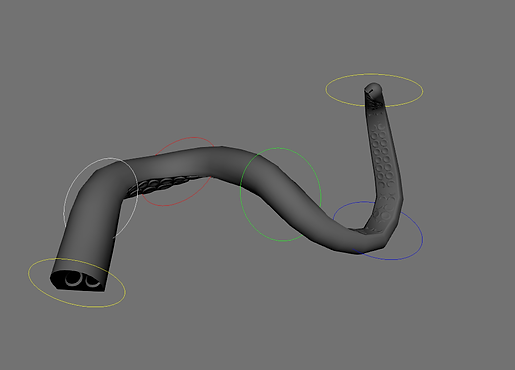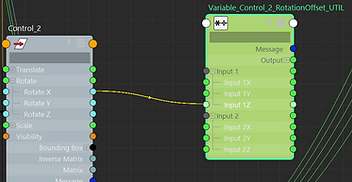###### For the Variable FK Rig, the goal was to be able to slide rotations and shapes along the structure of the tentacle. In order to be able to get this behavior, I had to solve the first problem: The sliding controls. After I solved that, I had to make those controls actually influence the joints along the appendage. Below I will detail my journey and the methods I used to build the rig I wanted.###### This will allow us to slide our control along the length of the rig, and give us the beginnings of a Variable FK structure.###### The first step is to constrain the control to every joint using a point constraint. Make sure to maintain offset here and also have your transformations frozen on the control beforehand. This will keep any nasty values from affecting our sliding.###### Most of my work on this piece was done in the Node Editor, so I went ahead and laid out my graph.###### So our controls now slide around. That's nice. To make the rig actually functional, those controls have to actually affect the rotation of our rig. Usully you could use orient constraints in this case, however that would require much more setup to control the contributions and make it play nice with other controls. To skip all that, we will continue to use set driven keys, but in combination with Multiply/Divide node utilities.###### The first step is to drive the rotation of our rig with our control. We want to be able to rotate our control and have it rotate the rig along the same axis. In this example, our control rotates along the X axis while the joint structure rotates along its Z axis to achieve the same rotation. To fix this, we will use a multiply divide node to flip those rotations.###### X to Z, Z to X, and Y to Y. Pretty straightforward.###### Reverse the Z value, so that they rotate along the same axis. You can also increase these amounts from 1 if you want the rotation to be more powerful. You can decide that later if necessary.###### Using more multiply divide nodes, one for each joint, we then hook up our rotation offset into each of these nodes. This will allow us to use the second input in each node to control the strength of the rotation relative to the joint's influence.###### We then connect each of these multiply divide nodes into their corresponding joint's rotations. In summation, the control's rotation changes coordinates and gets flipped, then it gets passed into multiply divide nodes, with no secondary inputs (yet), then passed directly to the joint's rotations.###### If you rotate the control now, it should produce a similar result to the image; each joint will rotate with the control. This is not the desired effect. It is now time to manipulate the secondary inputs of those multiple divide nodes.###### Again using Set Driven Keys, we will key each secondary input to be either 0,0,0 or 1,1,1 depending on our Pos Along Chain variable. As before, I use steps of 10 to control the influence changes. This will make it line up perfectly with the point constraints.###### In order to have multiple controls affect the same appendage, we must average out all of their rotation influences, and pass that value to their rotations.###### Taking the multiply divide nodes from two control structures, I create an average node for each pair to blend their outputs.###### I then connect each average node to its joint's rotation (instead of just the output of single multiply divide nodes as we constructed earlier). Now we can have two controls affecting the same rig structure.